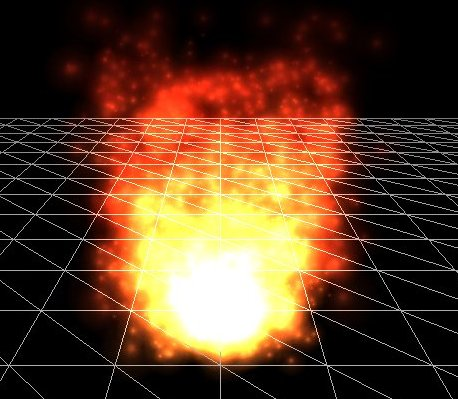# 粒子

Notestruct Particle {
glm::vec2 Position, Velocity;
glm::vec4 Color;
GLfloat Life;

Particle()
: Position(0.0f), Velocity(0.0f), Color(1.0f), Life(0.0f) { }
};


#version 330 core
layout (location = 0) in vec4 vertex; // <vec2 position, vec2 texCoords>

out vec2 TexCoords;
out vec4 ParticleColor;

uniform mat4 projection;
uniform vec2 offset;
uniform vec4 color;

void main()
{
float scale = 10.0f;
TexCoords = vertex.zw;
ParticleColor = color;
gl_Position = projection * vec4((vertex.xy * scale) + offset, 0.0, 1.0);
}


#version 330 core
in vec2 TexCoords;
in vec4 ParticleColor;
out vec4 color;

uniform sampler2D sprite;

void main()
{
color = (texture(sprite, TexCoords) * ParticleColor);
}


GLuint nr_particles = 500;
std::vector<Particle> particles;

for (GLuint i = 0; i < nr_particles; ++i)
particles.push_back(Particle());


GLuint nr_new_particles = 2;
for (GLuint i = 0; i < nr_new_particles; ++i)
{
int unusedParticle = FirstUnusedParticle();
RespawnParticle(particles[unusedParticle], object, offset);
}
// Update all particles
for (GLuint i = 0; i < nr_particles; ++i)
{
Particle &p = particles[i];
p.Life -= dt; // reduce life
if (p.Life > 0.0f)
{   // particle is alive, thus update
p.Position -= p.Velocity * dt;
p.Color.a -= dt * 2.5;
}
}


GLuint lastUsedParticle = 0;
GLuint FirstUnusedParticle()
{
// Search from last used particle, this will usually return almost instantly
for (GLuint i = lastUsedParticle; i < nr_particles; ++i){
if (particles[i].Life <= 0.0f){
lastUsedParticle = i;
return i;
}
}
// Otherwise, do a linear search
for (GLuint i = 0; i < lastUsedParticle; ++i){
if (particles[i].Life <= 0.0f){
lastUsedParticle = i;
return i;
}
}
// Override first particle if all others are alive
lastUsedParticle = 0;
return 0;
}


void RespawnParticle(Particle &particle, GameObject &object, glm::vec2 offset)
{
GLfloat random = ((rand() % 100) - 50) / 10.0f;
GLfloat rColor = 0.5 + ((rand() % 100) / 100.0f);
particle.Position = object.Position + random + offset;
particle.Color = glm::vec4(rColor, rColor, rColor, 1.0f);
particle.Life = 1.0f;
particle.Velocity = object.Velocity * 0.1f;
}


glBlendFunc(GL_SRC_ALPHA, GL_ONE);
for (Particle particle : particles)
{
if (particle.Life > 0.0f)
{
particleTexture.Bind();
glBindVertexArray(particleVAO);
glDrawArrays(GL_TRIANGLES, 0, 6);
glBindVertexArray(0);
}
}
glBlendFunc(GL_SRC_ALPHA, GL_ONE_MINUS_SRC_ALPHA);


ParticleGenerator   *Particles;

void Game::Init()
{
[...]
[...]
[...]
Particles = new ParticleGenerator(
ResourceManager::GetTexture("particle"),
500
);
}


void Game::Update(GLfloat dt)
{
[...]
// Update particles
Particles->Update(dt, *Ball, 2, glm::vec2(Ball->Radius / 2));
[...]
}


void Game::Render()
{
if (this->State == GAME_ACTIVE)
{
[...]
// Draw player
Player->Draw(*Renderer);
// Draw particles
Particles->Draw();
// Draw ball
Ball->Draw(*Renderer);
}
}